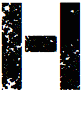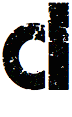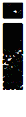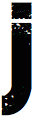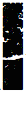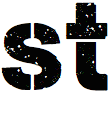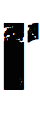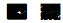## Movies from Thesis Work

### ECCV 00

Visualization of the learned model for walking. The learned body angles over one cycle have been used to animate a stick figure. The sequences below show (from left to right) the mean cycle, and the mean cycle plus the main modes of variation multiplied with gradually increased Gaussian noise (standard deviation 0.2, 0.5, 0.8 and 1.0).
Click on image for a movie of the sequence.

Following a person in 3D using the walking motion model. The distribution over body angles and location is represented by a discrete number of samples, and propagated in time with particle filtering. For each sample the image likelihood is evaluated at each time step (using motion, edge and ridge response). The white model projected in to the images is the expected value of the posterior distribution in each time step.
Click on image for a movie of the sequence.

### ICCV 01

Following arms with the general model of constant velocity. The distribution over body angles and location is represented by a discrete number of samples, and propagated in time with particle filtering. For each sample the image likelihood is evaluated at each time step (using motion, edge and ridge response). The white model projected in to the images is the expected value of the posterior distribution in each time step.
Click on image for a movie of the sequence.

### ECCV 02

Synthesizing activities using the example based motion model. Below, from left to right, movies of synthesized running (far left), walking (middle left), swing dance (middle right), and changing activity (far right) are shown. Color change of the head indicates that the sequence, from which the motion is selected, changes.
Click on image for a movie of the sequence.

Following an arm using the example based motion model (left). A fairly low number of particles are used. To compare, the arm is also followed with the smooth motion model described in (Sidenbladh et al., ICCV 01). With the same low number of particles, the smooth motion model loses track when the arm accelerates (middle), while the example based model handles these accelerations. However, with a significantly higher number of samples, the tracking with the smooth motion model is successful (right).
Click on image for a movie of the sequence.